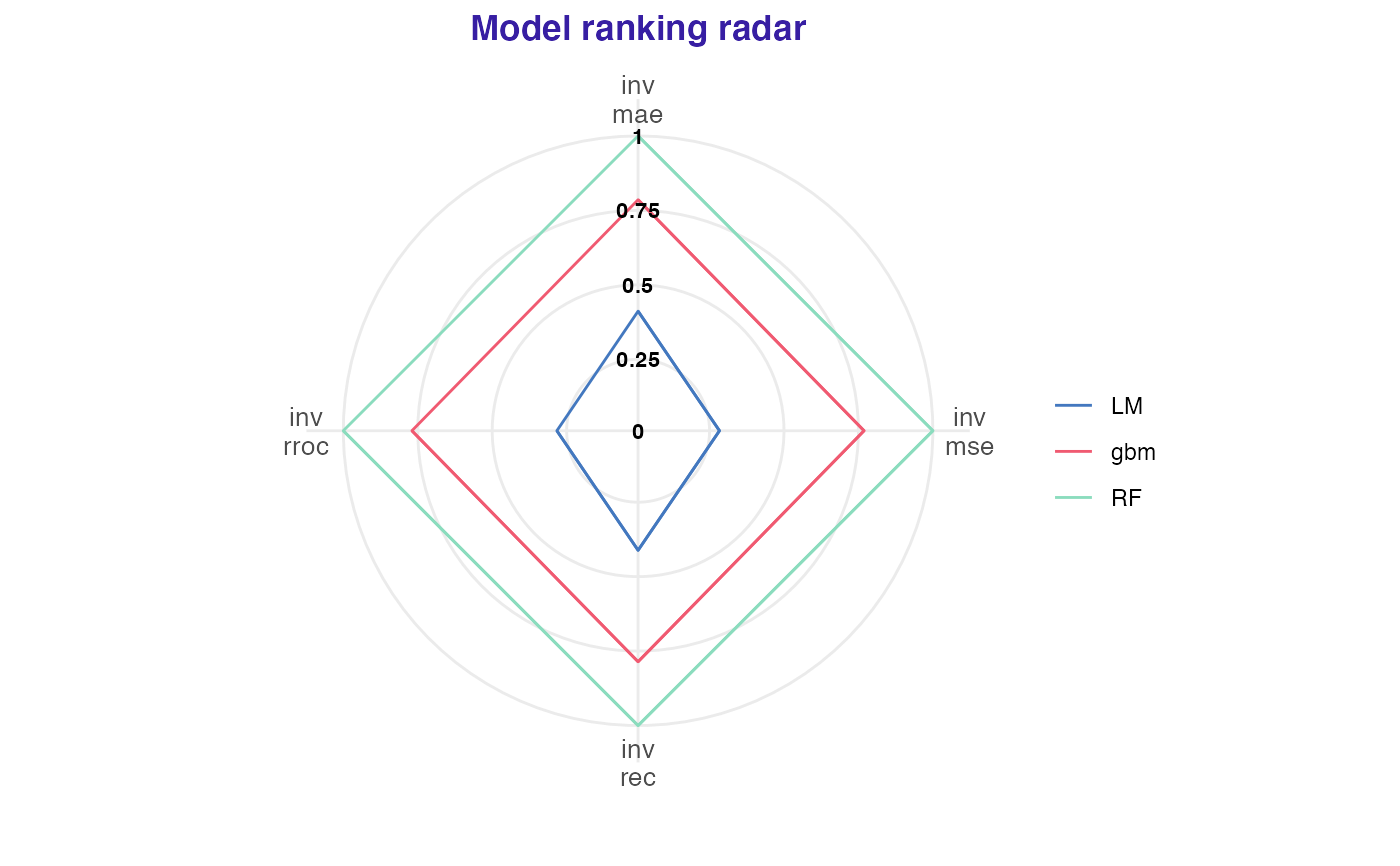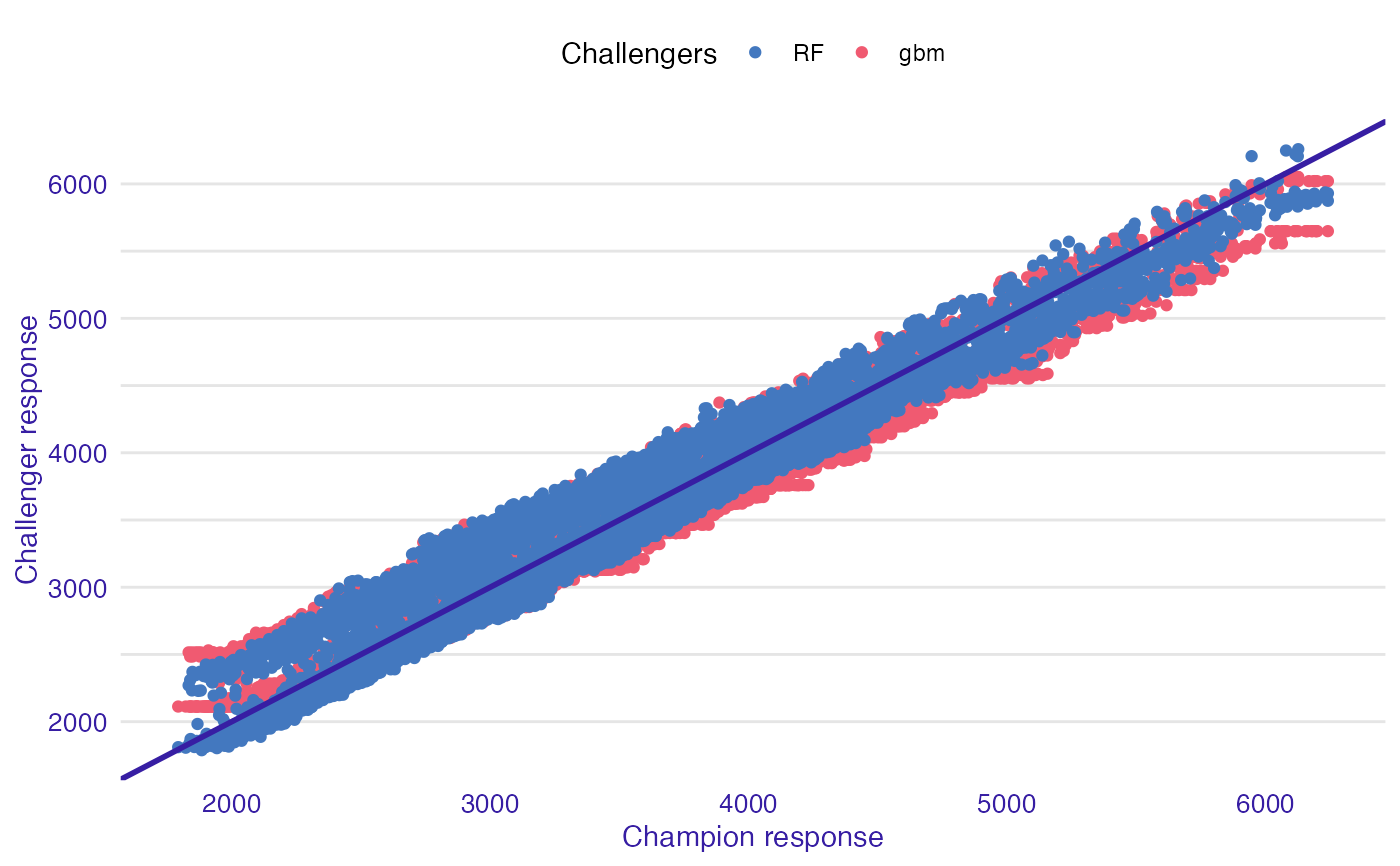The function creates objects that present global model performance using various measures. Those date can be easily plotted with plot function. It uses auditor package to create model_performance of all passed explainers. Keep in mind that type of task has to be specified.

overall_comparison(champion, challengers, type)

## Arguments

champion

- explainer of champion model.

challengers

- explainer of challenger model or list of explainers.

type

- type of the task. Either classification or regression

## Value

An object of the class overall_comparison It is a named list containing following fields:

• radar list of model_performance objects and other parameters that will be passed to generic plot function

• accordance data.frame object of champion responses and challenger's corresponding to them. Used to plot accordance.

• models_info data.frame containing information about models used in analysis

## Examples

# \donttest{
library("DALEXtra")
library("mlr")
id = "R",
data = apartments,
target = "m2.price"
)
learner_lm <- mlr::makeLearner(
"regr.lm"
)
model_lm <- mlr::train(learner_lm, task)
explainer_lm <- explain_mlr(model_lm, apartmentsTest, apartmentsTest$m2.price, label = "LM") #> Preparation of a new explainer is initiated #> -> model label : LM #> -> data : 9000 rows 6 cols #> -> target variable : 9000 values #> -> predict function : yhat.WrappedModel will be used ( default ) #> -> predicted values : No value for predict function target column. ( default ) #> -> model_info : package mlr , ver. 2.19.0 , task regression ( default ) #> -> predicted values : numerical, min = 1792.597 , mean = 3506.836 , max = 6241.447 #> -> residual function : difference between y and yhat ( default ) #> -> residuals : numerical, min = -257.2555 , mean = 4.687686 , max = 472.356 #> A new explainer has been created! learner_rf <- mlr::makeLearner( "regr.ranger" ) model_rf <- mlr::train(learner_rf, task) explainer_rf <- explain_mlr(model_rf, apartmentsTest, apartmentsTest$m2.price, label = "RF")
#> Preparation of a new explainer is initiated
#>   -> model label       :  RF
#>   -> data              :  9000  rows  6  cols
#>   -> target variable   :  9000  values
#>   -> predict function  :  yhat.WrappedModel  will be used (  default  )
#>   -> predicted values  :  No value for predict function target column. (  default  )
#>   -> model_info        :  package mlr , ver. 2.19.0 , task regression (  default  )
#>   -> predicted values  :  numerical, min =  1787.508 , mean =  3504.632 , max =  6258.499
#>   -> residual function :  difference between y and yhat (  default  )
#>   -> residuals         :  numerical, min =  -542.5728 , mean =  6.891499 , max =  758.0745
#>   A new explainer has been created!

learner_gbm <- mlr::makeLearner(
"regr.gbm"
)
model_gbm <- mlr::train(learner_gbm, task)
explainer_gbm <- explain_mlr(model_gbm, apartmentsTest, apartmentsTest$m2.price, label = "gbm") #> Preparation of a new explainer is initiated #> -> model label : gbm #> -> data : 9000 rows 6 cols #> -> target variable : 9000 values #> -> predict function : yhat.WrappedModel will be used ( default ) #> -> predicted values : No value for predict function target column. ( default ) #> -> model_info : package mlr , ver. 2.19.0 , task regression ( default ) #> -> predicted values : numerical, min = 2112.394 , mean = 3501.82 , max = 6051.511 #> -> residual function : difference between y and yhat ( default ) #> -> residuals : numerical, min = -509.3936 , mean = 9.703829 , max = 696.808 #> A new explainer has been created! data <- overall_comparison(explainer_lm, list(explainer_gbm, explainer_rf), type = "regression") plot(data) #>$radar_plot#>
#> \$accordance_plot#>
# }